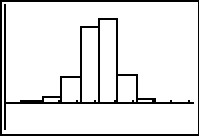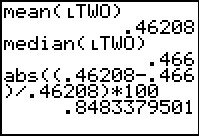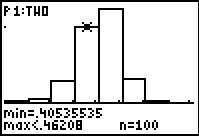••• ##### Device
• TI-83 Plus Family
• TI-84 Plus
• TI-84 Plus Silver Edition
•TI-84 Plus C Silver Edition
•TI-84 Plus CE
• ##### Software

TI Connect™
TI Connect™ CE

• ##### Report an Issue

Statistics: Assessing Normalcy
by Texas Instruments#### Overview

Students use four criteria to determine if a data set is normal. They begin by looking at a histogram to determine if it is symmetric and bell-shaped.

#### Key Steps

•In this activity, students will use four criteria to determine if a data set is normal. They begin by looking at a histogram to determine if it is symmetric and bell-shaped.

•Second, students compare the mean and the median and look at the percent difference to see if they are relatively the same.

•Third, students scale the histogram so each bar represents one standard deviation. They use the graph to record the number of data points in each standard deviation. The calculation of the percentage of points in each standard deviation will verify the 68-95-99.7 rule.